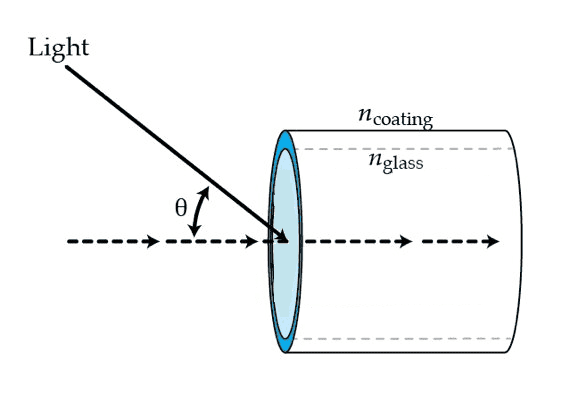# Internal reflection equation question

GatoGordo
As you can see we have 3 media here. Only focus on the glass and coating medium. Assume an incident ray comes from the air medium and is refracted inside the glass and then it is refracted again in the coating medium. The x angle is the angle inside the glass medium. In this case, if the incident ray,from the air, enters the glass it will create a refracted ray with an angle. This is the x angle. Now, let's say I want the refraction angle of the coating medium to be 90°. Why do I have to subtract 90° from the x angle to achieve this?

nglasssin(90°−x)=ncoatingsin(90°)Last edited by a moderator:

Gold Member
Look at your equation, without the $90^\circ$ shift, you'll have a negative answer, i.e. a negative index of refraction.

Homework Helper
It's because of the way the angles are defined. Angle of incidence and angle of refraction are the angles relative to the surface normal. That picture defines ##\theta## as relative to the horizontal, which is tangent to the surface. The angle of incidence is ##90^\circ - \theta##.

Gold Member
Summary:: Why do we subtract 90° from the incident angle when we want to find at which angle causes a refraction of 90° in a second medium?

Why do I have to subtract 90°
Your angle θ in the diagram needs to be modified to be the angle between the ray and the Normal to the surface (i.e. 90-θ ). It's the angle from the normal of the surface that is what's in the standard version of Snell's Law, which gives you the Critical Angle.